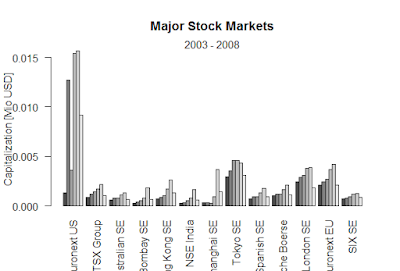Showing posts with label Breusch-Godfrey test. Show all posts
Showing posts with label Breusch-Godfrey test. Show all posts

## Breusch-Godfrey test for first-order serial correlation R

The following objects are masked from ‘package:base’:

as.Date, as.Date.numeric

> x <- rep(c(1, -1), 50)
> y1 <- 1 + x + rnorm(100)
>
> Perform Breusch-Godfrey test for first-order serial correlation:
Error: unexpected symbol in "Perform Breusc
> bgtest(y1 ~ x)

Breusch-Godfrey test for serial correlation of
order up to 1

data:  y1 ~ x
LM test = 2.402, df = 1, p-value = 0.1212

### > for fourth-order serial correlation

Error: unexpected symbol in "for fourth"
> bgtest(y1 ~ x, order = 4)

Breusch-Godfrey test for serial correlation of
order up to 4

data:  y1 ~ x
LM test = 10.869, df = 4, p-value = 0.02808

> bgtest(y1 ~ x, order = 6)

Breusch-Godfrey test for serial correlation of
order up to 6

data:  y1 ~ x
LM test = 12.851, df = 6, p-value = 0.04546

> Compare with Durbin-Watson test results:
Error: unexpected symbol in "Compare with"
> dwtest(y1 ~ x)

#### Durbin-Watson test

data:  y1 ~ x
DW = 2.295, p-value = 0.9443
alternative hypothesis: true autocorrelation is greater than 0

> y2 <- filter(y1, 0.5, method = "recursive")
> bgtest(y2 ~ x)

Breusch-Godfrey test for serial correlation of
order up to 1

data:  y2 ~ x
LM test = 15.039, df = 1, p-value = 0.0001053

> bg4 <- bgtest(y2 ~ x, order = 4)
> bg4

Breusch-Godfrey test for serial correlation of
order up to 4

data:  y2 ~ x
LM test = 20.162, df = 4, p-value = 0.0004638
Conclusion
> coeftest(bg4)

z test of coefficients:

Estimate Std. Error z value Pr(>|z|)
(Intercept)   0.0033737  0.1026701  0.0329 0.973787
x            -0.0016900  0.1026174 -0.0165 0.986860
lag(resid)_1  0.3875120  0.1013209  3.8246 0.000131
lag(resid)_2  0.1129702  0.1073329  1.0525 0.292560
lag(resid)_3 -0.1970706  0.1075560 -1.8323 0.066913
lag(resid)_4  0.1925046  0.1041504  1.8483 0.064554

(Intercept)
x
lag(resid)_1 ***
lag(resid)_2
lag(resid)_3 .
lag(resid)_4 .
---
Signif. codes:
0 ‘***’ 0.001 ‘**’ 0.01 ‘*’ 0.05 ‘.’ 0.1 ‘ ’ 1

### Black-Scholes formula-R

Black-Scholes formula-R > BlackScholes <- function(TypeFlag = c("c", "p"), S, X, Time, r, b, sigma) { TypeFla...